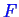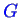Next: Phase Changes Up: Continuum Thermodynamics Previous: The Second Law

## The Gibbs Free Energy and Helmholtz Free Energy

The entropy gives a maximal principle, but for the entire universe. It would be useful to find other functions that have similar extremal properties but for a subsystem of the universe. For example, one doesn't usually state that ice will freeze at a particular temperature and pressure because the entropy of the ice plus the freezer plus the wires that run to the power station plus the power station, etc, have a total sum entropy that increases. Rather, it is decidedly more useful to have some other quantity that applies to the water/ice itself as a function of temperature and pressure of its environment.

The quintessential concept in the application of the laws of thermodynamics is how to derive that useful quantity from the second law. Their derivation depend on what aspects of the material can vary--in other words, a precise description of the experiment that would be performed on the material in which you are interested. The two most famous such quantities are the Gibbs Free Energy and Helmholtz Free energies.

The entropy allows us to define two new extensive thermodynamic variables: the Helmholtz free energy,, is the maximum amount of work a system can do a constant volume and temperature; the Gibbs free energyis the maximum amount of work a system can do at constant pressure and temperature.is a minimum for a closed systems at equilibrium with a fixed temperature and pressure.

Why define all these different functions--can't we just have one? No, because in practice, systems have different constraints imposed on them, and to understand them we need to find the physical principles that describe their behavior.Next: Phase Changes Up: Continuum Thermodynamics Previous: The Second Law
W. Craig Carter 2002-09-05